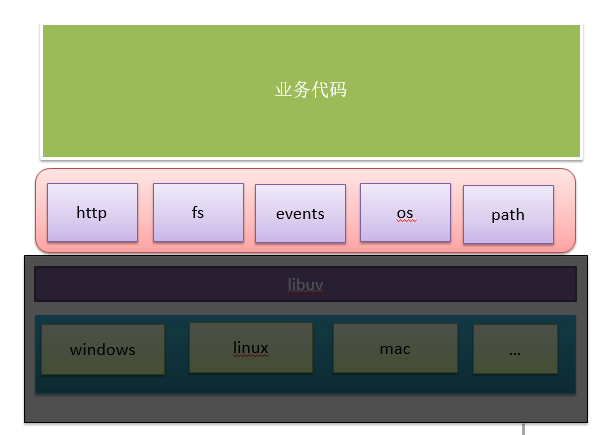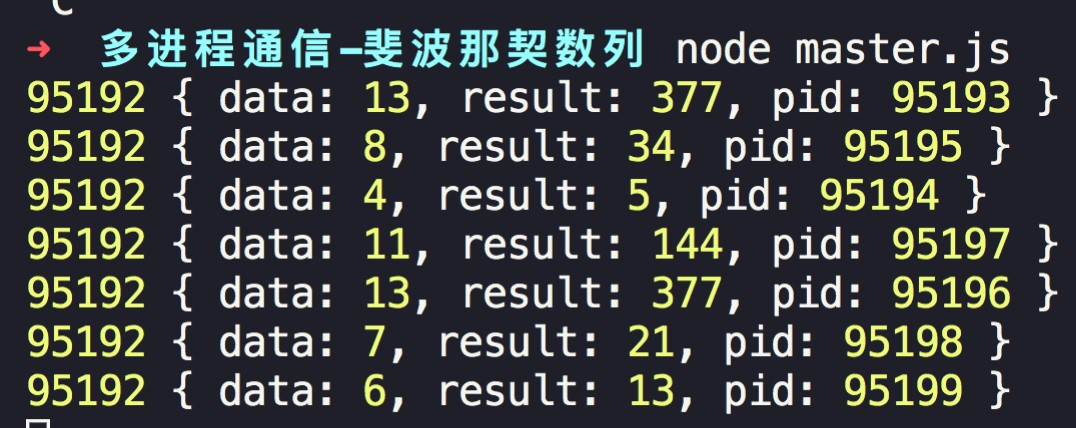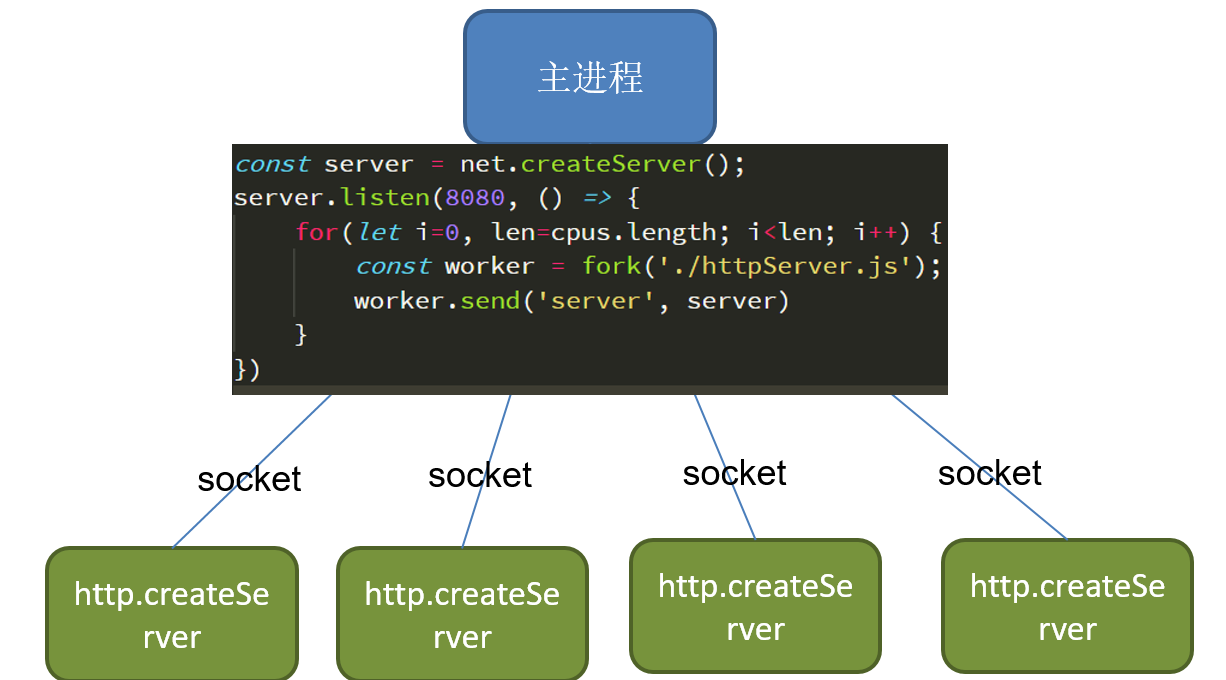# node多进程的创建与守护

node是单线程运行，我们的node项目如何利用多核CPU的资源，同时提高node服务的稳定性呢？

1. node的单线程

2. node多进程的创建

3. 多进程间的通信

4. 多进程的维护

### 1. node的单线程

Node是基于V8引擎之上构建的，决定了他与浏览器的机制很类似。• 这个线程挂掉后整个程序就会挂掉；

• 无法充分利用多核资源### 2. node多进程的创建

node中有提供`child_process`模块，这个模块中，提供了多个方法来创建子进程。

`COPYJAVASCRIPT`const { spawn, exec, execFile, fork } = require('child_process');``

```COPYJAVASCRIPT```// worker.js
const fib = (num) => {
if (num === 1 || num === 2) {
return num;
}
let a = 1, b = 2, sum = 0;
for (let i = 3; i <= num; i++) {
sum = a + b;
a = b;
b = sum;
}
return sum;
}

const num = Math.floor(Math.random() * 10) + 3;
const result = fib(num);
console.log(num, result, process.pid); // process.pid表示当前的进程id``````

spawnspawn('node', ['worker.js'])启动一个字进程来执行命令
execexec('node worker.js', (err, stdout, stderr) => {})启动一个子进程来执行命令，有回调
execFileexexFile('worker.js')启动一个子进程来执行可执行的文件
（头部要添加#!/usr/bin/env node）
forkfork('worker.js')与spawn类似，不过这里只需要自定js文件模块即可

```COPYJAVASCRIPT```const { fork } = require('child_process');
const cpus = require('os').cpus();

for(let i=0, len=cpus.length; i<len; i++) {
fork('./worker.js');
}``````

### 3. 多进程之间的通信

node中进程的通信主要在主从（子）进程之间进行通信，子进程之间无法直接通信，若要相互通信，则要通过主进程进行信息的转发。

• master.js：用来创建子进程和子进程间的通信；

• fib.js：计算斐波那契数列；

• log.js：输出斐波那契数列计算的结果；

```COPYJAVASCRIPT```// master.js

const { fork } = require('child_process');
const cpus = require('os').cpus();

const logWorker = fork('./log.js');

for(let i=0, len=cpus.length-1; i<len; i++) {
const worker = fork('./fib.js');
worker.send(Math.floor(Math.random()*10 + 4)); // 要计算的num
worker.on('message', (data) => { // 计算后返回的结果
logWorker.send(data); // 将结果发送给输出进程
})
}``````

```COPYJAVASCRIPT```// fib.js
const fib = (num) => {
if (num===1 || num===2) {
return num;
}
let a=1, b=2, sum=0;
for(let i=3; i<num; i++) {
sum = a + b;
a = b;
b = sum;
}
return sum;
}
process.on('message', num => {
const result = fib(num);

process.send(JSON.stringify({
num,
result,
pid: process.pid
}))
})``````

```COPYJAVASCRIPT```process.on('message', data => {
console.log(process.pid, data);
})`````````COPYJAVASCRIPT```// httpServer.js
const http = require('http');

http.createServer((req, res) => {
'Content-Type': 'text/html'
});
res.end(Math.random()+'');
}).listen(8080);
console.log('http server has started at 8080, pid: '+process.pid);``````

`COPYSHELL`Error: listen EADDRINUSE: address already in use :::8080`````COPYJAVASCRIPT```// master.js
const fork = require('child_process').fork;
const net = require('net');

const server = net.createServer();
const child1 = fork('./httpServer1.js'); // random
const child2 = fork('./httpServer2.js'); // now

server.listen(8080, () => {
child1.send('server', server);
child2.send('server', server);
server.close();
})``````

httpServer1.js:

```COPYJAVASCRIPT```const http = require('http');

const server = http.createServer((req, res) => {
'Content-Type': 'text/plain'
});
res.end(Math.random()+', at pid: ' + process.pid);
});

process.on('message', (type, tcp) => {
if (type==='server') {
tcp.on('connection', socket => {
server.emit('connection', socket)
})
}
})``````

httpServer2.js:

```COPYJAVASCRIPT```const http = require('http');

const server = http.createServer((req, res) => {
'Content-Type': 'text/plain'
});
res.end(Date.now()+', at pid: ' + process.pid);
});

process.on('message', (type, tcp) => {
if (type==='server') {
tcp.on('connection', socket => {
server.emit('connection', socket)
})
}
})``````

### 4. 多进程的守护

```COPYJAVASCRIPT```const fork = require('child_process').fork;
const cpus = require('os').cpus();
const net = require('net');

const server = net.createServer();

const createServer = () => {
const worker = fork('./httpServer.js');
worker.on('exit', () => {
// 当有进程退出时，则创建一个新的进程
console.log('worker exit: ' + worker.pid);
createServer();
});

worker.send('server', server);
console.log('create worker: ' + worker.pid);
}

server.listen(8080, () => {
for(let i=0, len=cpus.length; i<len; i++) {
createServer();
}
})``````

#### cluster模块

```COPYJAVASCRIPT```const cluster = require('cluster');
const http = require('http');
const cpus = require('os').cpus();

if (cluster.isMaster) {
console.log(`主进程 \${process.pid} 正在运行`);

// 衍生工作进程。
for (let i = 0, len=cpus.length; i < len; i++) {
cluster.fork();
}

cluster.on('exit', (worker) => {
console.log(`工作进程 \${worker.process.pid} 已退出`);
cluster.fork();
});
} else {
http.createServer((req, res) => {
res.end(Math.random()+ ', at pid: ' + process.pid);
}).listen(8080);

console.log(`工作进程 \${process.pid} 已启动`);
}``````

### 5. 总结

node虽然是单线程运行的，但我们可以通过创建多个子进程，来充分利用多核CPU资源，通过可以监听进程的一些事件，来感知每个进程的运行状态，来提高我们项目整体的稳定性。### 公众号：### 公众号：